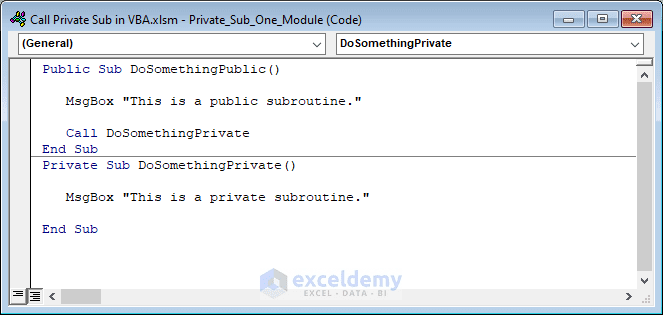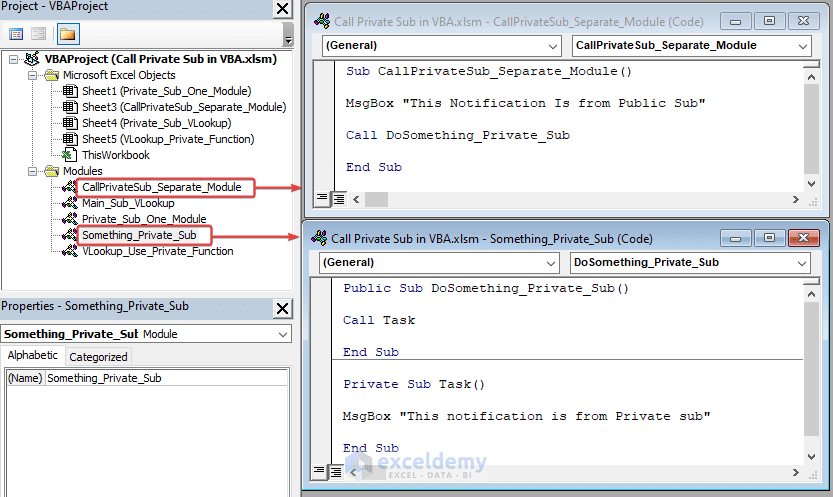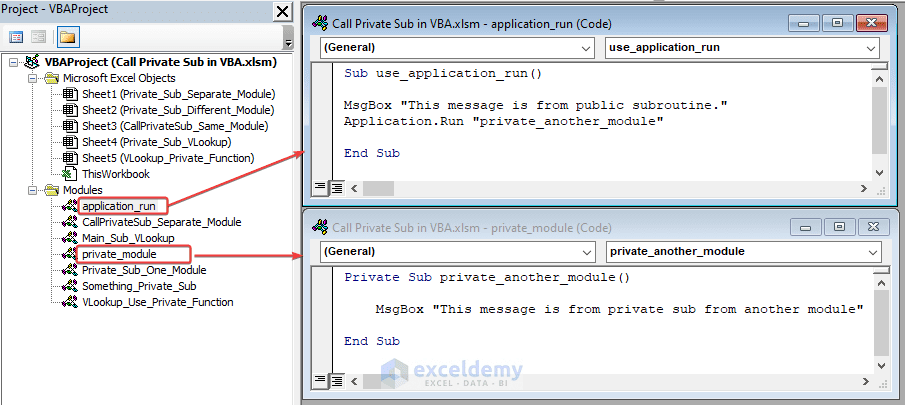# How to Call Private Sub in Excel VBA (4 Simple Ways)

Get FREE Advanced Excel Exercises with Solutions!

Visual Basic for Applications (VBA) is a programming language commonly used in Microsoft Excel, Word, and other Office applications. Calling a Sub or Private Sub is a quite beginning task to learn in VBA. In this article, we will discuss how to call a private sub in VBA and why it may be useful to do so. We will also explore some best practices and potential pitfalls to be aware of when using private subroutines in your VBA code.## What Is Private Sub in Excel VBA?

In Excel VBA, a Private Sub is a subroutine or method that can only be accessed from within the module or class where it is defined. Private Subs are not visible to other modules or classes in the same project, so they cannot be called or executed from outside their module or class.

Private Subs are often used to define helper functions or procedures that are specific to a particular module or class and not intended for use elsewhere. They can also be used to encapsulate certain functionality or logic within a module or class, making it easier to maintain and modify the code in the future.

## How to Call Private Sub in Excel VBA: 4 Simple Ways

In this section, we’ll know how to call private sub in vba. Also how to use a private sub in VBA code to do different tasks. We will explain with simple tasks. Firstly, you have to Open VBA Window and Insert New Module. Follow the link if you don’t know it already.

### 1. Call Private Sub in One Module of VBA to Show Different Notifications with MsgBox

In the first method, we will explain how to use private sub along with public sub in the same module. We will show two notifications that will come from public and private subs respectively. Let’s see the procedure.

• Write the following code in a new VBA module and run it.

Code:

``````Public Sub DoSomethingPublic()
MsgBox "This is a public subroutine."
Call DoSomethingPrivate
End Sub
Private Sub DoSomethingPrivate()
MsgBox "This is a private subroutine."
End Sub``````
• In the code, firstly the main sub shows a message with MsgBox and then, calls a private sub with its name. The private sub was also instructed to show another message.
• Consecutively, you will see notification comes from the main sub.• Finally, click OK and you will see the notification from the private sub.### 2. VBA Call  Private Sub from Another Module for Copying Values from Worksheet

In this method, we will discuss how to call a private sub from another module. It’s a bit tricky. It’s not directly possible to call a private sub directly from another module. So, we will call the public sub from another module which eventually uses the private sub from the same module.

• This time, insert two separate modules and write the attached codes there. One has the private sub in it.

Code (Public):

``````Sub CallPrivateSub_Separate_Module()
MsgBox "This Notification Is from Public Sub"
Call DoSomething_Private_Sub
End Sub``````

Code (Private):

``````Public Sub DoSomething_Private_Sub()
End Sub
MsgBox "This notification is from Private sub"
End Sub``````
• Lastly, run the public sub code and you will see a notification from the public sub first.• Simply, click OK and you will see the notification from the private sub from another module.In the code, we included the private sub in another public sub and then called that public sub from another sub in another module. It’s a bit critical. Also, we used simple MsgBox to express the result of the subs.

### 3. Use Application.Run to Call Private Sub from Another Module

There’s a simpler way to call a private sub from another module. This time, let’s use the Application.Run the command to use the private sub from another module.

• Firstly, Insert the following codes in separate modules and run the public sub.

Code (public):

``````Sub use_application_run()
MsgBox "This message is from public subroutine."
Application.Run "private_another_module"
End Sub``````

Code (private):

``````Private Sub private_another_module()
MsgBox "This message is from private sub from another module"
End Sub``````
In the public sub, we called the private sub with Application.Run command. In both the subs, we used the MsgBox as the tasks.
• Consecutively, you get a notification from the public sub first after running the code (public).• Later on, will have the notification from the private sub of another module.### 4. Call Private Sub to VLookup and Show Result on Worksheet

Now, we will vlookup at our dataset using the facilities of private sub. Let’s walk through the procedures. This time also, we will use both public and private sub in the same module.

• Firstly, create a dataset from where you want to vlookup.• Then, write the following code in a new module and run it.

Code:

``````Sub Main_Sub_VLookup()
Call Use_VLookup
End Sub
Private Sub Use_VLookup()
Dim lookup_value As Range
Dim table_array As Range
Dim col_index_num As Long
Dim range_lookup As Boolean
Dim result As Variant
' Define the lookup value
Set lookup_value = Worksheets("Private_Sub_VLookup").Range("C13")
' Define the table array
Set table_array = Worksheets("Private_Sub_VLookup").Range("B5:D11")
' Define the column index number
col_index_num = 3
' Define the range lookup option
range_lookup = False
' Call the VLOOKUP function and store the result in the 'result' variable
result = Application.WorksheetFunction.VLookup(lookup_value, table_array, col_index_num, range_lookup)
' Display the result in worksheet
Worksheets("Private_Sub_VLookup").Range("C14") = result
End Sub``````In the code, we have used comment’s for each segment, go through them to understand the code. This code defines a private sub Use_VLookup that performs a VLOOKUP operation on a table in the worksheet “Private_Sub_VLookup” and displays the result in cell C14. The private sub is called from a public sub named  Main_Sub_VLookup.
• After running the code, the vlookup result will appear on the worksheet.## How to Call Private Function in Excel VBA

So far, we have seen how to use private sub. Let’s see how to use a private function of VBA. We will vlookup at the dataset using a private function. Follow the described procedures.

• Firstly, create a dataset to vlookup from.• Now, insert the following code in a new module and run it.

Code:

``````Dim lookup_value As Range
Dim table_array As Range
Dim col_index_num As Long
Dim range_lookup As Boolean
Dim result As Variant
' Define the lookup value
Set lookup_value = Worksheets("VLookup_Private_Function").Range("C13")
' Define the table array
Set table_array = Range("B5:D11")
' Define the column index number
col_index_num = 3
' Define the range lookup option
range_lookup = False
' Call the private MyVLookup function and store the result in the 'result' variable
result = MyVLookup(lookup_value, table_array, col_index_num, range_lookup)
' Display the result in worksheet
Worksheets("VLookup_Private_Function").Range("C14") = result
End Sub
Private Function MyVLookup(lookup_value As Variant, table_array As Range, col_index_num As Long, range_lookup As Boolean) As Variant
' Call the VLOOKUP function and return the result
MyVLookup = Application.WorksheetFunction.VLookup(lookup_value, table_array, col_index_num, range_lookup)
End Function``````In the code, we have used the VLookup function in a private function. Then, called the private function from the public sub.
• As a result, you will see the vlookup result appear in the worksheet.• Why would you use a Private Sub in Excel VBA?

Ans: Private Subs are often used to define helper functions or procedures that are specific to a particular module or class and not intended for use elsewhere. They can also be used to encapsulate certain functionality or logic within a module or class, making it easier to maintain and modify the code in the future.

• Can Private Subs be called from outside their module or class?

Ans: No, you can access Private Subs only from within the module or class where you have defined them. If you want to call a Private Sub from outside its module or class, you need to define a separate public Sub or Function that calls the Private Sub.

• What are some best practices for using Private Subs in Excel VBA?

Ans: Some best practices for using Private Subs in Excel VBA include using them to encapsulate logic and functionality, giving them descriptive names that reflect their purpose, and using them to break up large blocks of code into smaller, more manageable pieces. It’s also a good idea to use error-handling techniques to handle unexpected errors and prevent your code from crashing.

## Things to Remember

• Don’t forget to save the file as an xlsm file before running any code.
• You can’t call a private sub from another module unless you have put it inside a public sub.
• Be careful about calling any sub by its name, not by the name of the module.

## Conclusion

Tags:Mehedi Hasan Shimul

Hi! I am Mehedi Hasan Shimul. As I am an Engineer solving different problems with the help of Excel amuses me. I write Excel related different problem solving articles here. Hope it will help you.

We will be happy to hear your thoughtsAdvanced Excel Exercises with Solutions PDF